# Given that the ASA (concentration of acetylsalicylic acid) is 0.0087 M, calculate the concentration of tetraaquasalicylato...

Given that the ASA (concentration of acetylsalicylic acid) is 0.0087 M, calculate the concentration of tetraaquasalicylato ion [Fe(H2O)4SA]+ in solution 1

Solution 1: volume = 2.00 mL, absorbance = 0.339

If you could show work that would be great! Thank you soo much!!

[H+] = 0.0087 M per liter

[H+] equal to tetraaquasalicylato ion at 2ml

so

the concentration of tetraaquasalicylato ion in 2ml = 0.0087/0.002 = 4.35 M

#### Earn Coin

Coins can be redeemed for fabulous gifts.

Similar Homework Help Questions
• ### Aspirin is acetylsalicylic acid (ASA) ( C9H8O4). It reacts with NaOH ( a base) to produce...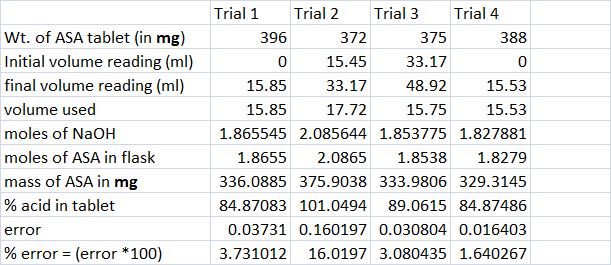Aspirin is acetylsalicylic acid (ASA) ( C9H8O4). It reacts with NaOH ( a base) to produce salt (sodium acetylate) and water. Both Aspirin and NaOH react in 1:1 molar ratio, as               C9H8O4 +         NaOH     →       NaC9H7O4     +        H2O So, moles of NaOH = moles of ASA Or, mmol of of NaOH = mmole of ASA           ; mmol is milli mol and mmole of NaOH = Molarity of NaOH * Volume of naOH used (in ml)                                   = (0.1177 M) *...

• ### II. Digestion of Acetylsalicylic acid(standard and tablet) with NaOH . Digestion of Acetylsalicylic Acid Standard to Pr...

II. Digestion of Acetylsalicylic acid(standard and tablet) with NaOH . Digestion of Acetylsalicylic Acid Standard to Prepare Stock Solution: Obtain ca. 0.1 g dried acetylsalicylic acid (MM = 180.16 g/mol), accurately weighed, and quantitatively transfer to a 125-ml Erlenmeyer flask. Add 5 ml 1.0 M NaOH and heat mixture to boiling on a hot plate. This will hydrolyze the acetylsalicylic acid to sodium salicylate. If necessary, rinse down the walls of the Erlenmeyer with DI water to ensure complete hydrolysis...

• ### 3a) The solubility of Aspirin (acetylsalicylic acid) at 25C is 3.0 mg/mL of water. Calculate the...

3a) The solubility of Aspirin (acetylsalicylic acid) at 25C is 3.0 mg/mL of water. Calculate the molar concentration (M) of acetylsalicylic acid in solution at this temperature. What density assumption can help you in solving this problem? b) The acid dissociation constant, Ka for acetylsalicylic acid is 3.0 x 10 -4. calculate the pH of this solution.

• ### Acid Over-titration : Calculate the acetate ion concentration?

Calculate the acetate ion concentration in a solution prepared bydissolving 3.80×10^-3 mol of HCl(g) in 1.00 L of 2.00 Maqueous acetic acid (Ka = 1.80×10^-5).Calculate the pH of the above solutionMy work :Ka = product/ reactant1.8 x 10^-5 = [CH3COOCl][H2O] / [HCl][CH3COOH]1.8 x 10^-5 = [CH3COOCl] / [3.8 x 10^-3 M][2M]CH3COOCl = 0.006But this is incorrect. What am I doing wrong? Thank you for yourtime!

• ### this is from the lab Spectrophometric determination of Aspirin content of tablets. How do find the concentration using...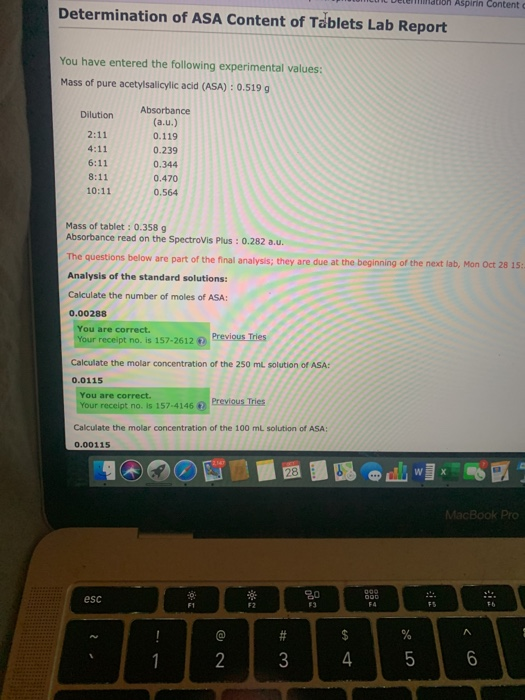this is from the lab Spectrophometric determination of Aspirin content of tablets. How do find the concentration using beers law? CUI Dele t on Aspirin Content Determination of ASA Content of Tablets Lab Report You have entered the following experimental values: Mass of pure acetylsalicylic acid (ASA) : 0.519 g Dilution 2:11 4:11 6:11 8:11 10:11 Absorbance (a.u.) 0.119 0.239 0.344 0.470 0.564 Mass of tablet : 0.3589 Absorbance read on the Spectrovis Plus : 0.282 a.u. The questions below...

• ### Using your data, calculate the moles and mass of acetylsalicylic acid in each tablet (molar mass ...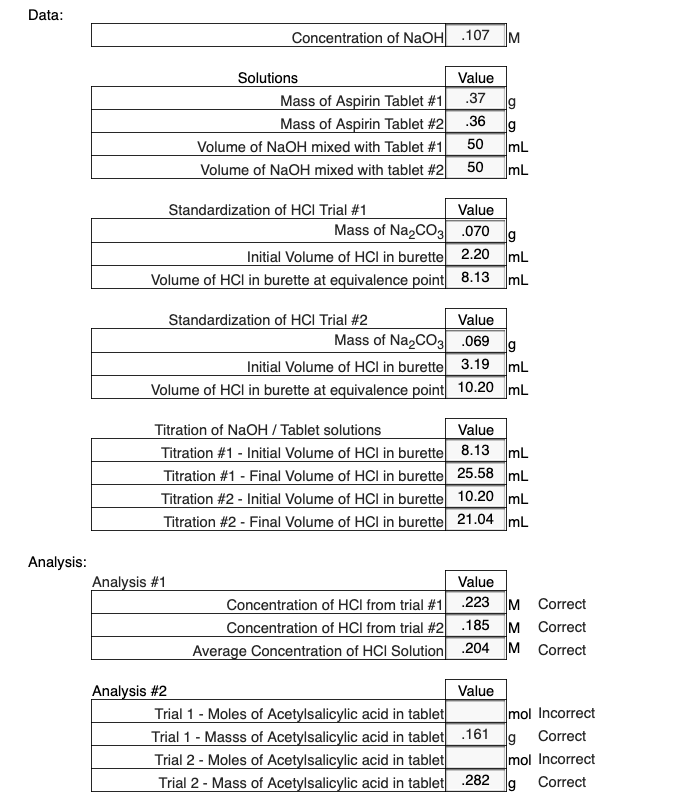Using your data, calculate the moles and mass of acetylsalicylic acid in each tablet (molar mass = 180.16 g/mol). Data Concentration of NaOH 107 M Solutions Mass of Aspirin Tablet #11 Mass of Aspirin Tablet #21 Volume of NaOH mixed with Tablet #1 | Volume of NaOH mixed with tablet #2 Value .37 .36 50 mL 50 mL Standardization of HCl Trial #1 Value Mass of Na2CO3 .070 a Initial Volume of HCI in burettel 2.20 mL Volume of HCl...

• ### You are given 75 mL of 0.70 M acetic acid/acetate buffer to test. The starting composition...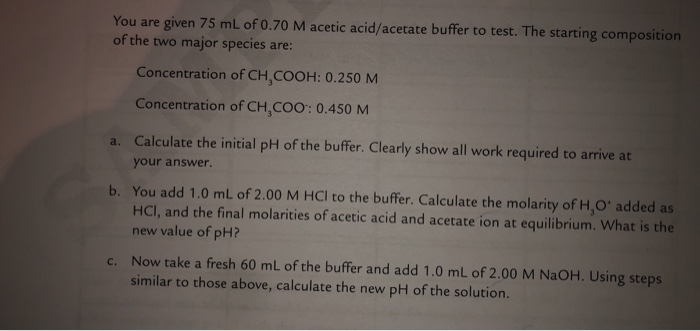You are given 75 mL of 0.70 M acetic acid/acetate buffer to test. The starting composition of the two major species are: Concentration of CH,COOH: 0.250 M Concentration of CH,COO: 0.450 M a. Calculate the initial pH of the buffer. Clearly show all work required to arrive at your answer. b. You add 1.0 mL of 2.00 M HCl to the buffer. Calculate the molarity of H, O' added as HCl, and the final molarities of acetic acid and acetate...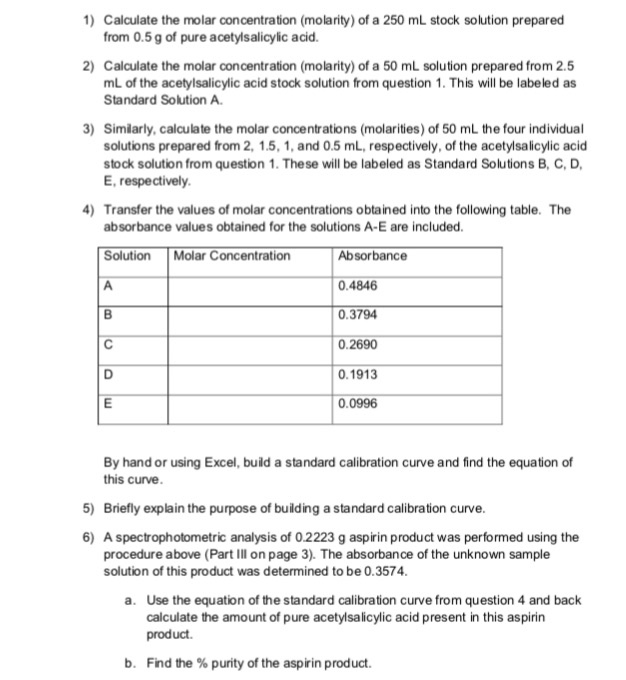Please help me answer questions from pre-lab homework Aspirin Analysis Lab 1) Calculate the molar concentration (molarity) of a 250 mL stock solution prepared from 0.5 g of pure acetylsalicylic acid. 2) Calculate the molar concentration (molarity) of a 50 mL solution prepared from 2.5 mL of the acetylsalicylic acid stock solution from question 1. This will be labeled as Standard Solution A. 3) Simlarly, calculate the molar concentrations (molarities) of 50 mL the four individual solutions prepared from 2,...

• ### The following information is given : Concentration (M) of starting solution: 0.024 % Transmittance, %T, (Blank)...

The following information is given : Concentration (M) of starting solution: 0.024 % Transmittance, %T, (Blank) : 98.6 % T (15-mL 0.024 M Cu2+ + 7 mL conc NH3 diluted with distilled H2O to 100 mL total volume):63.9 % T (15-mL Unknown Cu2+ solution + 7 mL conc NH3 diluted with distilled H2O to 100 mL total volume) :38.6 I need to find the following: Absorbance (blank) : Absorbance (15- mL Dilution ) : Ratio M/A (use 0.024 M as...

• ### 1. Calculate the pKa of lactic acid, given that when the concentration of lactic acid is...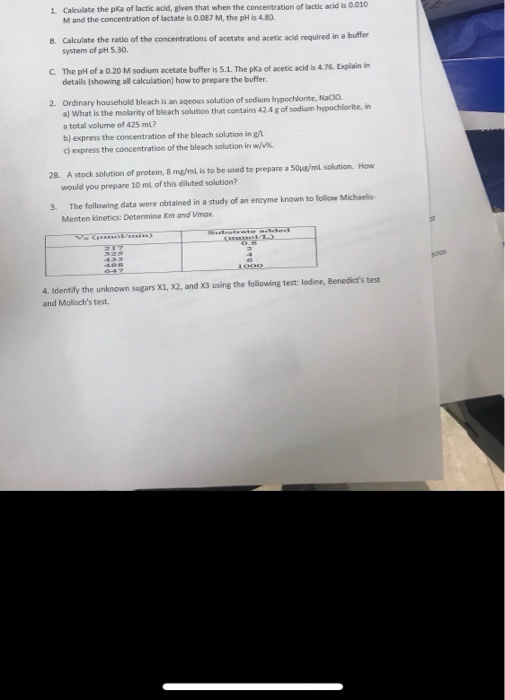1. Calculate the pKa of lactic acid, given that when the concentration of lactic acid is 0.010 M and the concentration of lactate is 0.087 M, the pH is 4.80 B. Calculate the ratio of the concentrations of acetate and acetic acid required in a buffer system of pH 5.30 C The pH of a 0.20 M sodium acetate buffer is 5.1. The pKa of acetic acid is 4.76. Explain in details (showing all calculation) how to prepare the buffer...